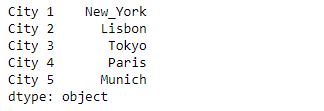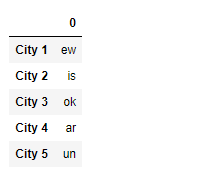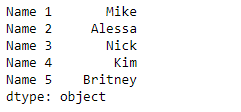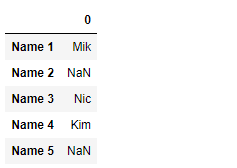Open In App

# Python | Pandas Series.str.extract()

`Series.str` can be used to access the values of the series as strings and apply several methods to it. Pandas` Series.str.extract()` function is used to extract capture groups in the regex pat as columns in a DataFrame. For each subject string in the Series, extract groups from the first match of regular expression pat.

Syntax: Series.str.extract(pat, flags=0, expand=True)

Parameter :
pat : Regular expression pattern with capturing groups.
flags : int, default 0 (no flags)
expand : If True, return DataFrame with one column per capture group.

Returns : DataFrame or Series or Index

Example #1: Use `Series.str.extract()` function to extract groups from the string in the underlying data of the given series object.

 `# importing pandas as pd``import` `pandas as pd`` ` `# importing re for regular expressions``import` `re`` ` `# Creating the Series``sr ``=` `pd.Series([``'New_York'``, ``'Lisbon'``, ``'Tokyo'``, ``'Paris'``, ``'Munich'``])`` ` `# Creating the index``idx ``=` `[``'City 1'``, ``'City 2'``, ``'City 3'``, ``'City 4'``, ``'City 5'``]`` ` `# set the index``sr.index ``=` `idx`` ` `# Print the series``print``(sr)`

Output :Now we will use `Series.str.extract()` function to extract groups from the strings in the given series object.

 `# extract groups having a vowel followed by``# any character``result ``=` `sr.``str``.extract(pat ``=` `'([aeiou].)'``)`` ` `# print the result``print``(result)`

Output :As we can see in the output, the `Series.str.extract()` function has returned a dataframe containing a column of the extracted group.

Example #2 : Use `Series.str.extract()` function to extract groups from the string in the underlying data of the given series object.

 `# importing pandas as pd``import` `pandas as pd`` ` `# importing re for regular expressions``import` `re`` ` `# Creating the Series``sr ``=` `pd.Series([``'Mike'``, ``'Alessa'``, ``'Nick'``, ``'Kim'``, ``'Britney'``])`` ` `# Creating the index``idx ``=` `[``'Name 1'``, ``'Name 2'``, ``'Name 3'``, ``'Name 4'``, ``'Name 5'``]`` ` `# set the index``sr.index ``=` `idx`` ` `# Print the series``print``(sr)`

Output :Now we will use `Series.str.extract()` function to extract groups from the strings in the given series object.

 `# extract groups having any capital letter``# followed by 'i' and any other character``result ``=` `sr.``str``.extract(pat ``=` `'([A-Z]i.)'``)`` ` `# print the result``print``(result)`

Output :As we can see in the output, the `Series.str.extract()` function has returned a dataframe containing a column of the extracted group.